×

Search anything:

# Latent Semantic Analysis for text summarization

#### Machine Learning (ML)Get this book -> Problems on Array: For Interviews and Competitive Programming

Reading time: 40 minutes | Coding time: 10 minutes

Latent Semantic Analysis is an robust Algebric-Statistical method which extracts hidden semantic structures of words and sentences i.e. it extracts the features that cannot be directly mentioned. These features are essential to data , but are not original features of the dataset. It is an unsupervised approach along with the usage of Natural Language Processing(NLP).

NLP + Unsupervised Learning

It is an efficient technique in order to abstract out the hidden context of the document.

## Benefits of LSA

1. Reduce the dimensionality of the original text-based dataset.
2. It helps us understand what each topic is encoding.
3. Analyze word association in text corpus.
4. Find relations between terms.
5. LSA has been used to assist in performing prior art searches for patents.

## Derivation

Let X be a matrix where element (i,j) describes the occurrence of term i in document j (this can be, for example, the frequency). X will look like this: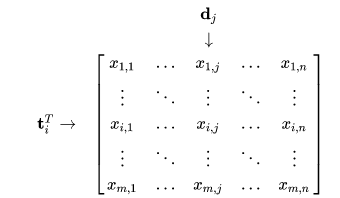Now a row in this matrix will be a vector corresponding to a term, giving its relation to each document: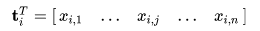Likewise, a column in this matrix will be a vector corresponding to a document, giving its relation to each term: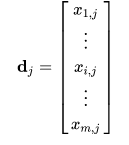Now the dot product between two term vectors gives the correlation between the terms over the set of documents. The matrix product XXT contains all these dot products and hence giving their correltion.
Now, there exists a decomposition of X such that U and V are orthogonal metrices. This is called Singular Matrix decomposition: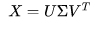The matrix products giving us the term and document correlations then become: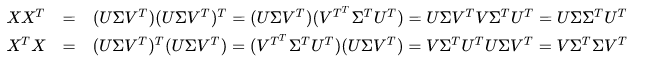Both products have the same non-zero eigenvalues, now the decomposition looks like this: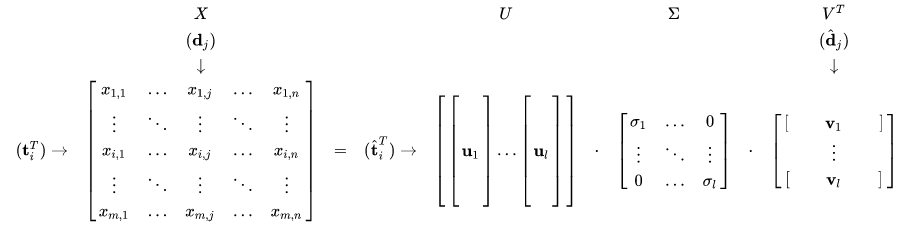The values of sigma's are called singular values and ui and vi are called the left and right singular vectors. Matrix VT will denote the strength of each word in a sentence or document. The use of this matrix will become clear as we will proceed towards the implementation. The Document vector dj is an approximation in the lower-dimensional space.We write this approximation as: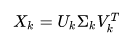## Algorithm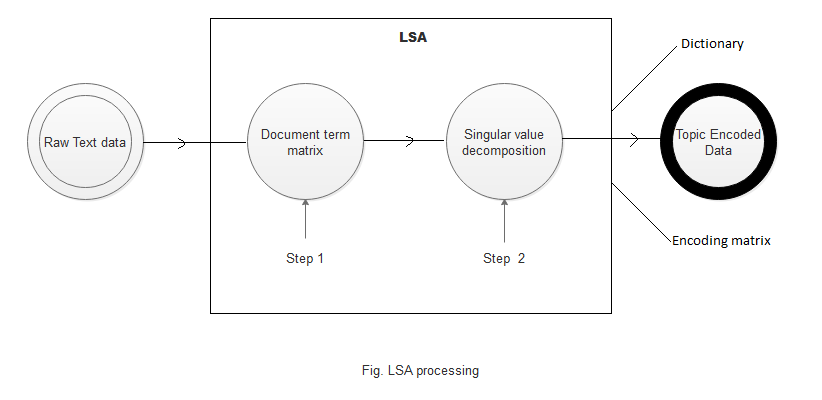The algorithm for LSA consists of three major steps:

1. Input matrix creation: The input document is represented as a matrix to understand and perform calculations on it. Thus, a document term matrix is generated.The cells are used to represent the importance of words in sentences.
Different approaches can be used for filling out the cell values. There are different approaches to fill out the cell values. These approaches are as follows.
• Frequency of word: the cell is filled in with the frequency of the word in the sentence.
• Binary representation: the cell is filled in with 0/1 depending on the existence of a word in the sentence.
• Tf-Idf (Term Frequency-Inverse Document Frequency): the cell is filled in with tf-idf value of the word. Higher tf-idf value means that the word is more frequent in the sentence but less frequent in the whole document. The higher value indicates that the word is much more representative for that sentence than others.
• Log entropy: the cell is filled in with log-entropy value of the word, which gives information on how informative the word is in the sentence.
• Root type: the cell is filled in with frequency of the word if its root type is a noun, otherwise the cell value is set to 0.

2. Singular Value decomposition(SVD): In this step we perform the singular value decomposition on the generated document term matrix. SVD is an algebraic method that can model relationships among words/phrases and sentences. The basic idea behind SVD is that document term matrix can be represented as points in Euclidean space known as vectors. These vectors are used to display the documents or sentences in our case in this space. Besides having the capability of modelling relationships among words and sentences, SVD has the capability of noise reduction, which helps to improve accuracy.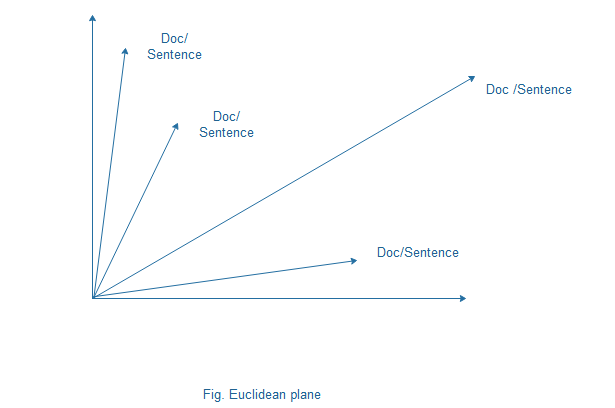1. Sentence Selection : Using the results of SVD different algorithms are used to select important sentences. Here we have used Topic method to extract concepts and sub-concepts from the SVD calculations and are called topics of the input document. These topics can be sub-topics, and then the sentences are collected from the main topics.

High number of common words among sentences indicates that the sentences are semantically related. Meaning of a sentence is decided using the word it contains, and meaning of words are decided using the sentences that contains the word.

Note- Dictionary and Encoding matrix are the by-products obtained during the execution of LSA processing.Dictionary is set of all words that occur once atleast in our document. While the encoded data represents words of our sentences in terms of their indivisual strengths. This strength helps us determine the exact affect of each word in out sentence/document.

### Limitations

LSA has several limitations:

The first one is that it does not use the information about word order, syntactic relations, and morphologies. This kind of information can be necessary for finding out the meaning of words and texts.

The second limitation is that it uses no world knowledge, but just the information that exists in input document.

The third limitation is related to the performance of the algorithm. With larger and more inhomogeneous data the performance decreases sharply. The decrease in performance is caused by SVD which is a very complex algorithm.

## Implementation

Let us consider a document containing following sentences:

``````"An intern at OpenGenus",
"Developer at OpenGenus",
"A ML intern",
"A ML developer"
``````

It can be represented as Document-term matrix (Sentences in our case):

Sentences/Words AN AT INTERN OPENGENUS DEVELOPER ML
An intern at OpenGenus 1 0 1 1 0 0
Developer at OpenGenus 0 1 0 1 1 0
A ML intern 1 0 1 0 0 1
An ML developer 1 0 0 0 1 1
• We have used binary data representation method to represent data in matrix.
• Where each row represents one of our documents,here one of three sentence fragments.
• Each column represents a word from the dictionary that shows atleast one of the four documents.

Each sentence will be represented in the corresponding vector form as follows:
"An intern at OpenGenus" = (1,0,1,1,0,0)

Using step 2 of algorithm we reduce the dimensionality of original dataset by unicoding it using latent features.

## Pseudocode

We use python libraries pandas and numpy for data visualization and manipulation. sklearn library is used to process the data.

``````doc = [
"An intern at OpenGenus",
"Developer at OpenGenus",
"A ML intern",
"A ML developer"
]

from sklearn.feature_extraction.text import CountVectorizer

# Converting each document into an vector
vectorizer = CountVectorizer()
bag_of_words = vectorizer.fit_transform(doc)
``````

Document is converted into a vectorized bag of words.
Bag of words - The bag-of-words model is a simplifying representation used in natural language processing and information retrieval (IR). In this model, a text (such as a sentence or a document) is represented as the bag (multiset) of its words, disregarding grammar and even word order but keeping multiplicity.

``````#look at the vectors
bag_of_words.todense()
``````
``````matrix([[1, 1, 0, 1, 0, 1],
[0, 1, 1, 0, 0, 1],
[0, 0, 0, 1, 1, 0],
[0, 0, 1, 0, 1, 0]], dtype=int64)
``````
``````#Singular value decomposition
from sklearn.decomposition import TruncatedSVD
#This process encodes our original data into topic encoded data
svd = TruncatedSVD(n_components = 2)
lsa = svd.fit_transform(bag_of_words)

#Using pandas to look at the output of lsa
import pandas as pd

topic_encoded_df = pd.DataFrame(lsa, columns=["topic1", "topic2"])
topic_encoded_df["doc"]= doc
display(topic_encoded_df[["doc","topic1","topic2"]])
``````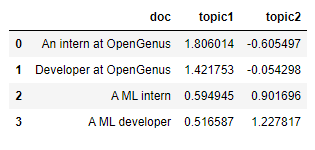This encoded matrix gives us better understanding of what each topic represents. It also represents how the sentence 1 and sentence 2 are more about OpenGenus while sentence 3 and 4 are more about machine learning as it represents the strength value for each topic. Here our main topics represented are OpenGenus and Machine Learning(ML).

``````#A look at dictionary
dictionary = vectorizer.get_feature_names()
dictionary
``````

Output:

``````['an', 'at', 'developer', 'intern', 'ml', 'opengenus']
``````
``````encoding_matrix=pd.DataFrame(svd.components_,index["topic1","topic2"],columns=dictionary).T
encoding_matrix
#numerical values can be thought of as an expression of that word in respective topic
``````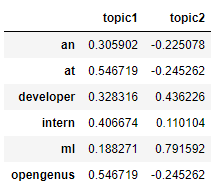``````#Considering the words affecting variance in data
import numpy as np
encoding_matrix['abs_topic1']=np.abs(encoding_matrix["topic1"])
encoding_matrix['abs_topic2']=np.abs(encoding_matrix["topic2"])
encoding_matrix.sort_values('abs_topic1',ascending=False)
``````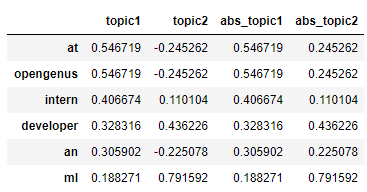``````#We need the matrix with absolute values only to determine the strength of each part of sentence effectively
final_matrix=encoding_matrix.sort_values('abs_topic1',ascending=False)
final_matrix[["abs_topic1","abs_topic2"]]
``````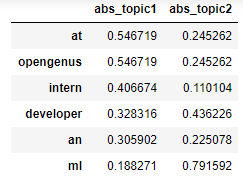``````#Extracting out final sentence from topic 1
sentence1= final_matrix[final_matrix["abs_topic1"]>=0.4]
sentence1[['abs_topic1']]
``````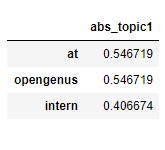Thus the obtained sentence will be Intern at OpenGenus.

``````#Extracting out final sentence from topic 2
sentence2=final_matrix[final_matrix["abs_topic2"]>=0.4]
sentence2[['abs_topic2']]
``````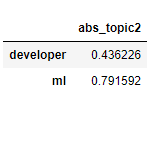Thus we get second sentence as ML Developer.

Negative words have same affect as positive words and have the same affect. Thus should be treated equally.
If we consider sentences as documents/concepts in more practical approach, then using the above data we can easily extract out concepts with more strength for our summarization.

The above pseudocode gives an insight to LSA processing and data visualization.

## Conclusion

We learned about the Latent Semantic Analysis(LSA) for text summarization and implemented a python code to visualize its working on a predefined document. Finally we obtained following information using LSA:

1. "Intern at OpenGenus".
2. "ML Developer".#### Ashutosh Vashisht

B.Tech (IT) Student at Amity School of Engineering and Technology

Latent Semantic Analysis for text summarization